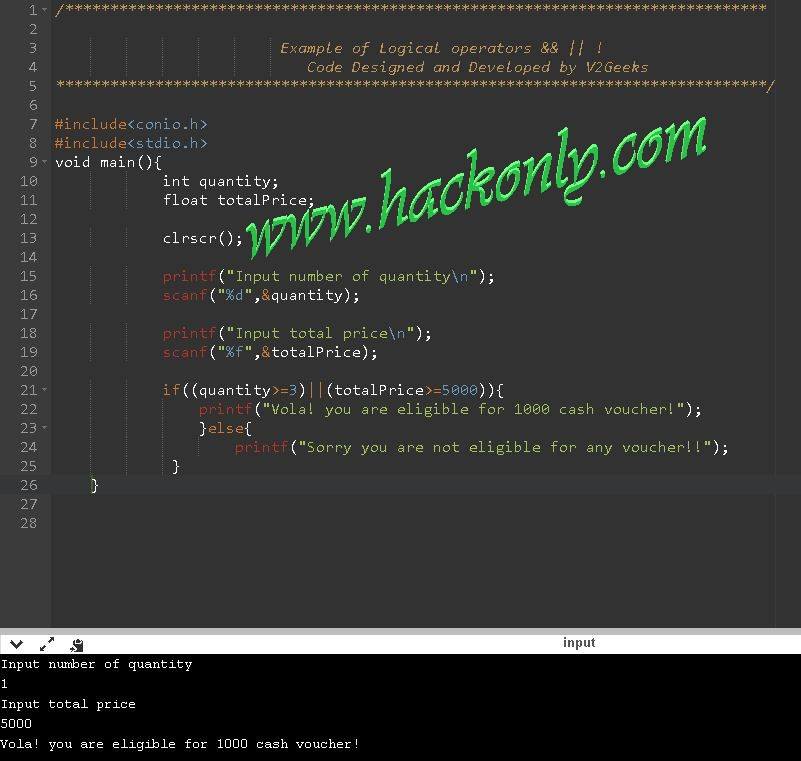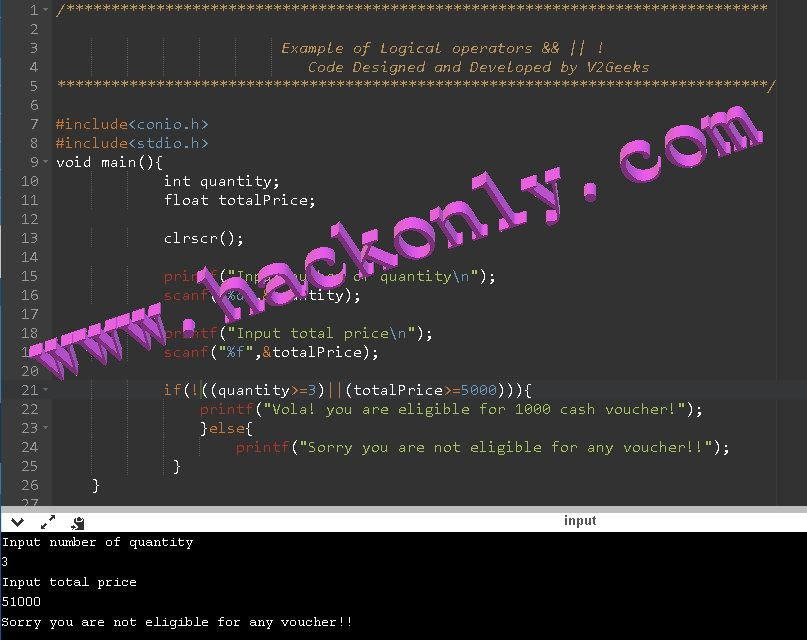# Field Manual for Hackers

## Example of Logical Operators in C

Logical Operators
An operator used to perform Boolean logic upon two Boolean expressions is called Logical operator. Logical operators return Boolean results (true or false) and take Boolean values as operands.C has three logical operators.  &&(AND), ||(OR), !(NOT)

Note: ! has lowest priority, while && has highest.

The logical operators && and || are used when we want to test more than one condition and make decisions. An Example is
a > b && c = =10

A Logical expression as shown  above yields a value of one or zero according to the truth table shown below.Truth Table
If a =20, b=10 and c=10 than previous logical results in True as 1&& 1 yields 1 following above truth table for AND.

AND Example
In below C program, the 'if' condition becomes true, if and only if both, quantity is greater or equal to 3 and at the same time total cart price is greater or equals to 5000.AND(&&) Example

OR Example
In below C program, the 'if' condition becomes true, if either side of OR operator yields to true.OR (|| ) Example
NOT Example
In below C program you can clearly see, ! operator reverses the decision of logical expression as we read in Truth table before.NOT(!) Example